•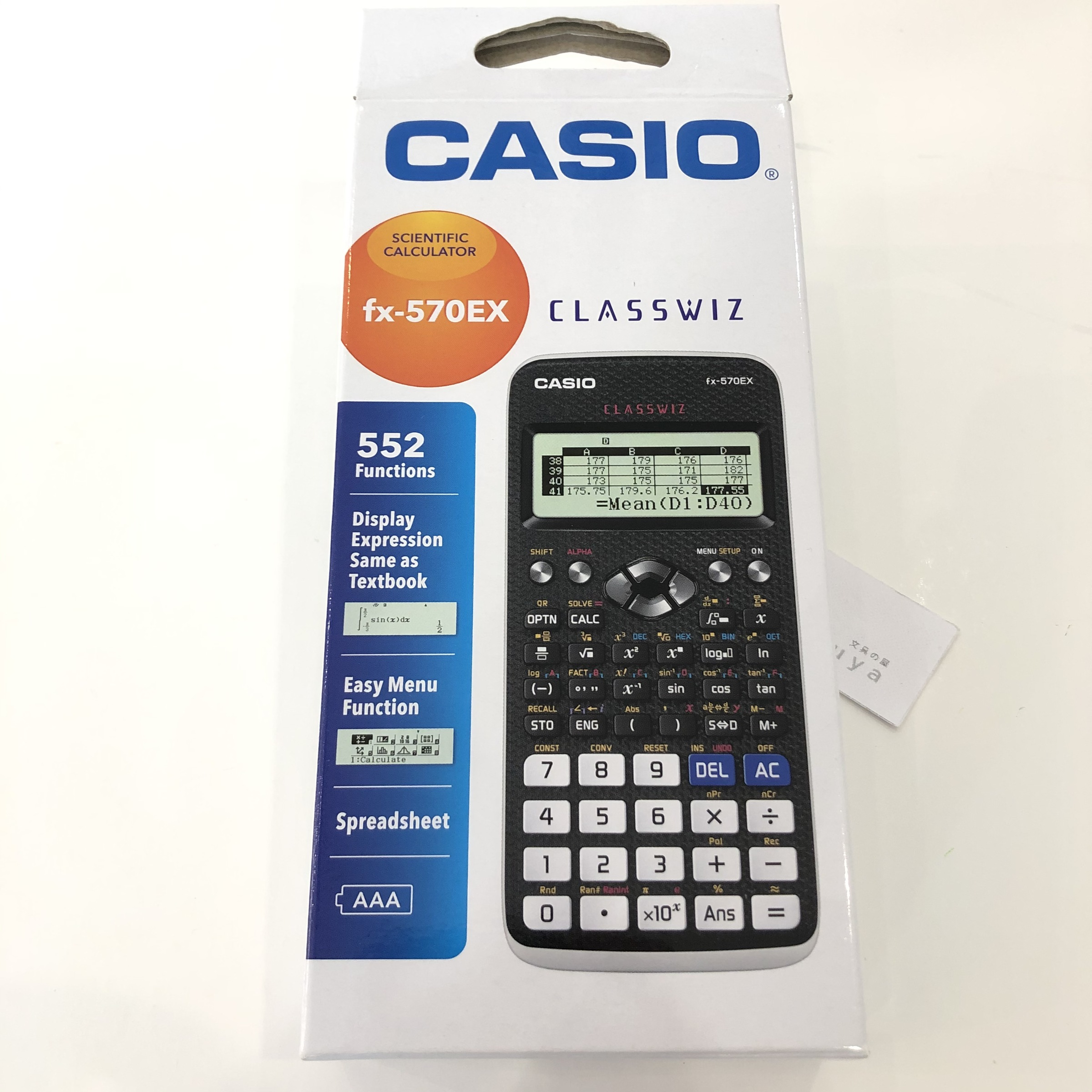•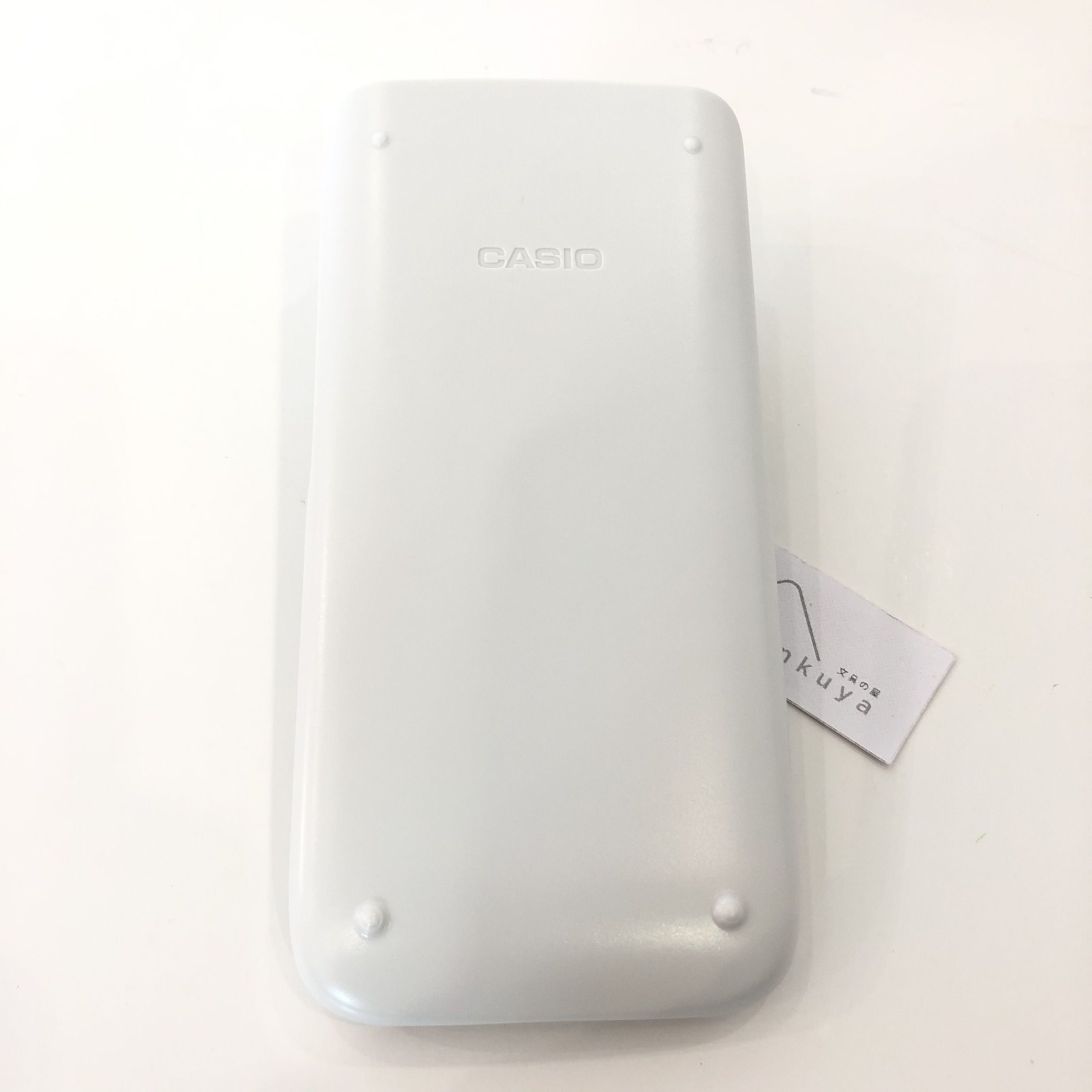•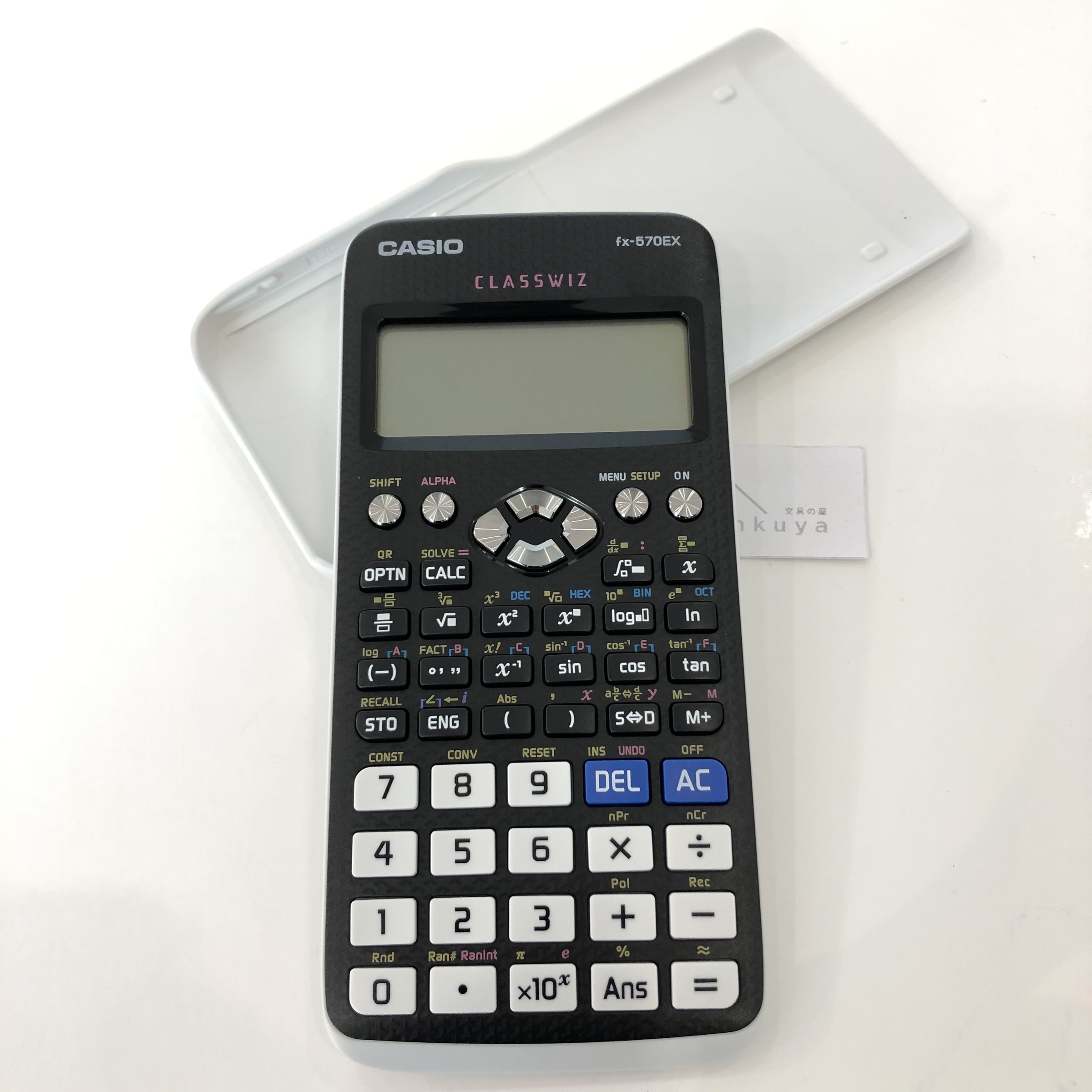••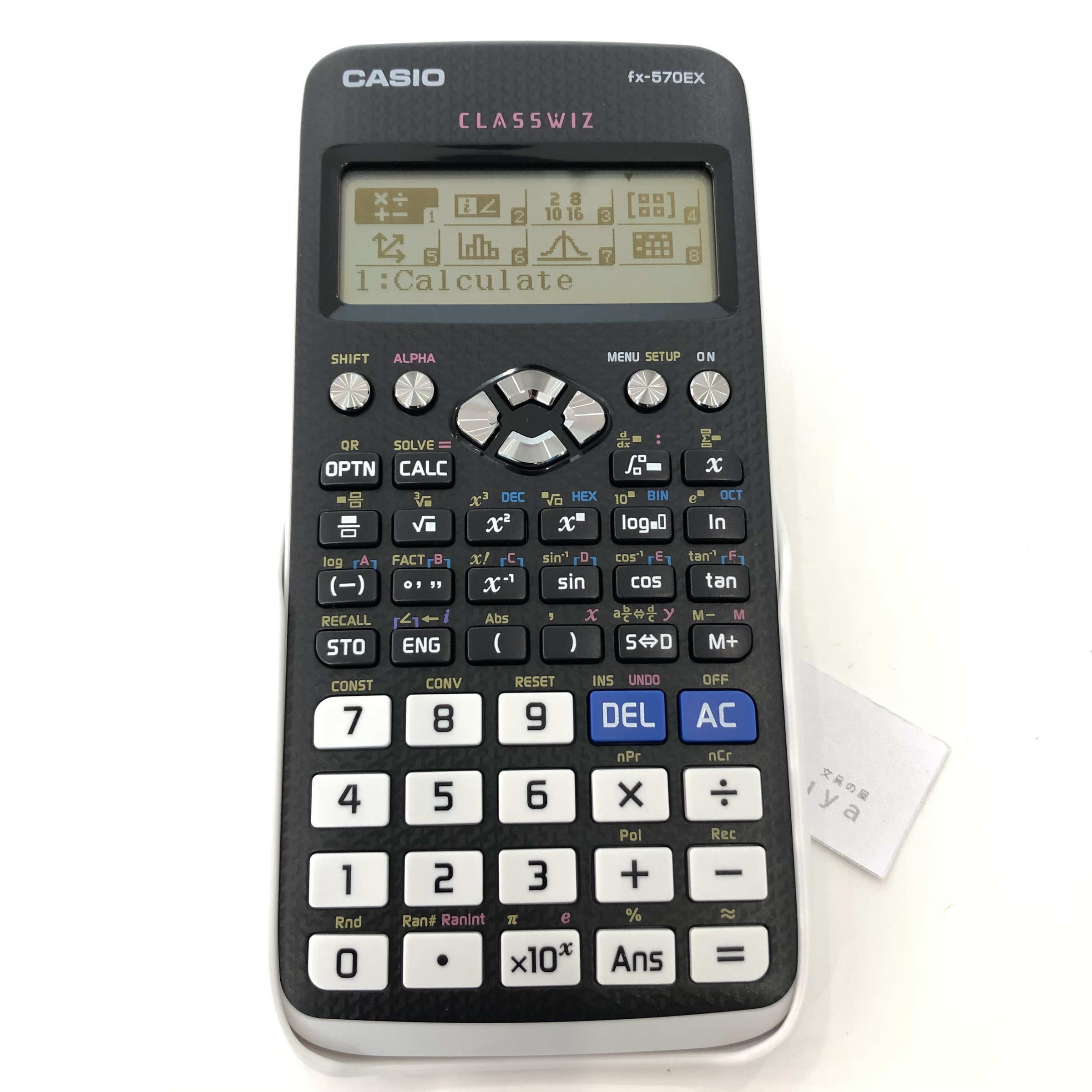•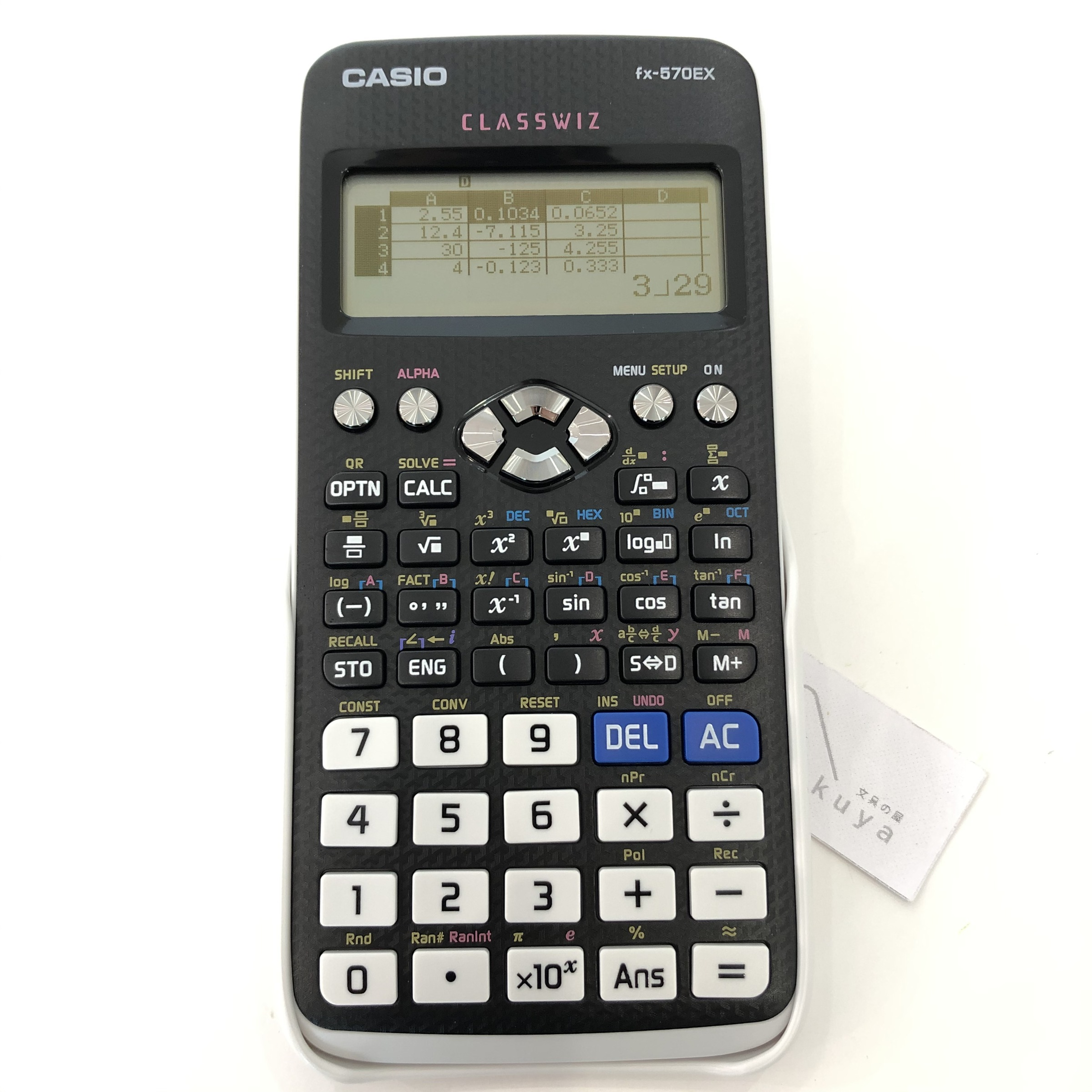•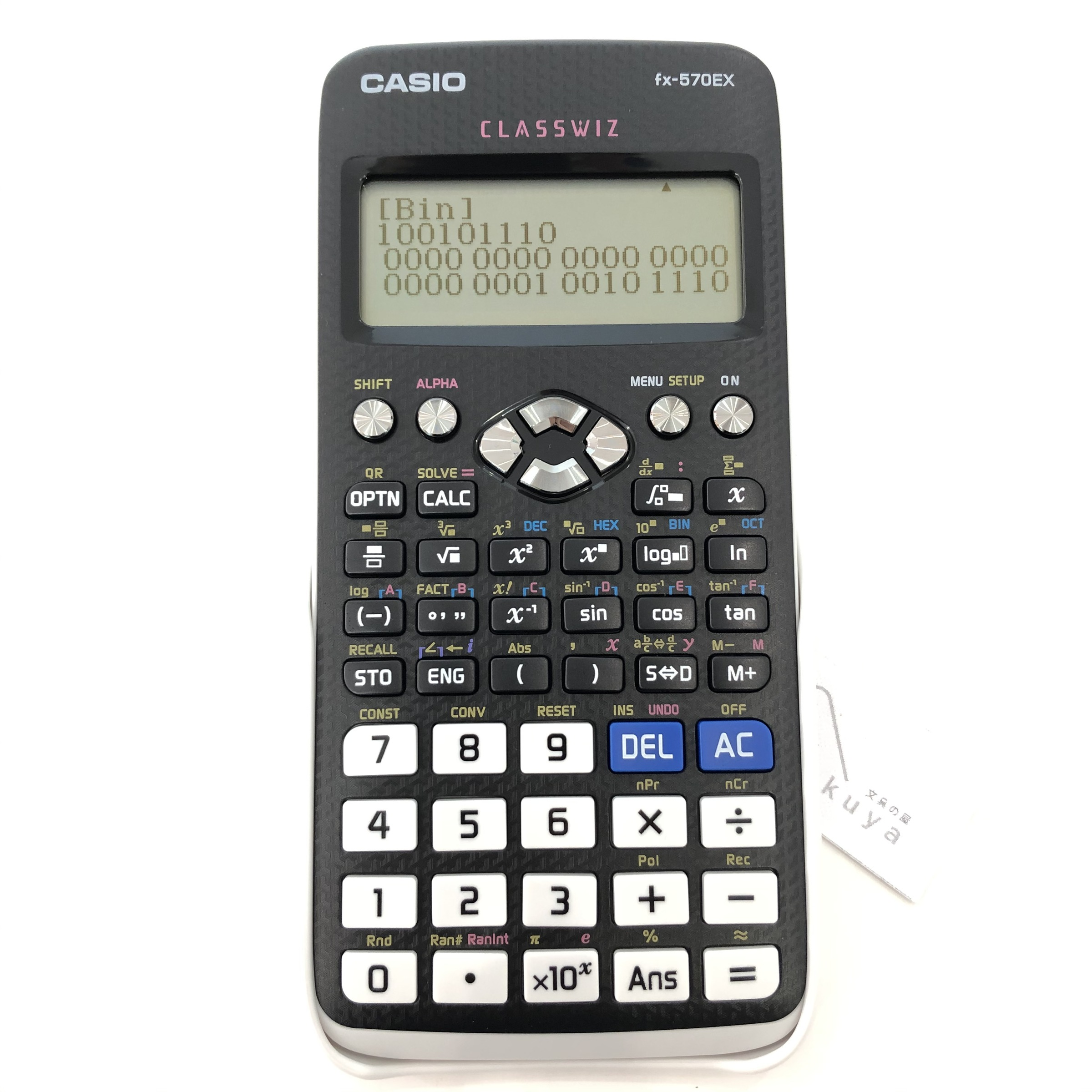•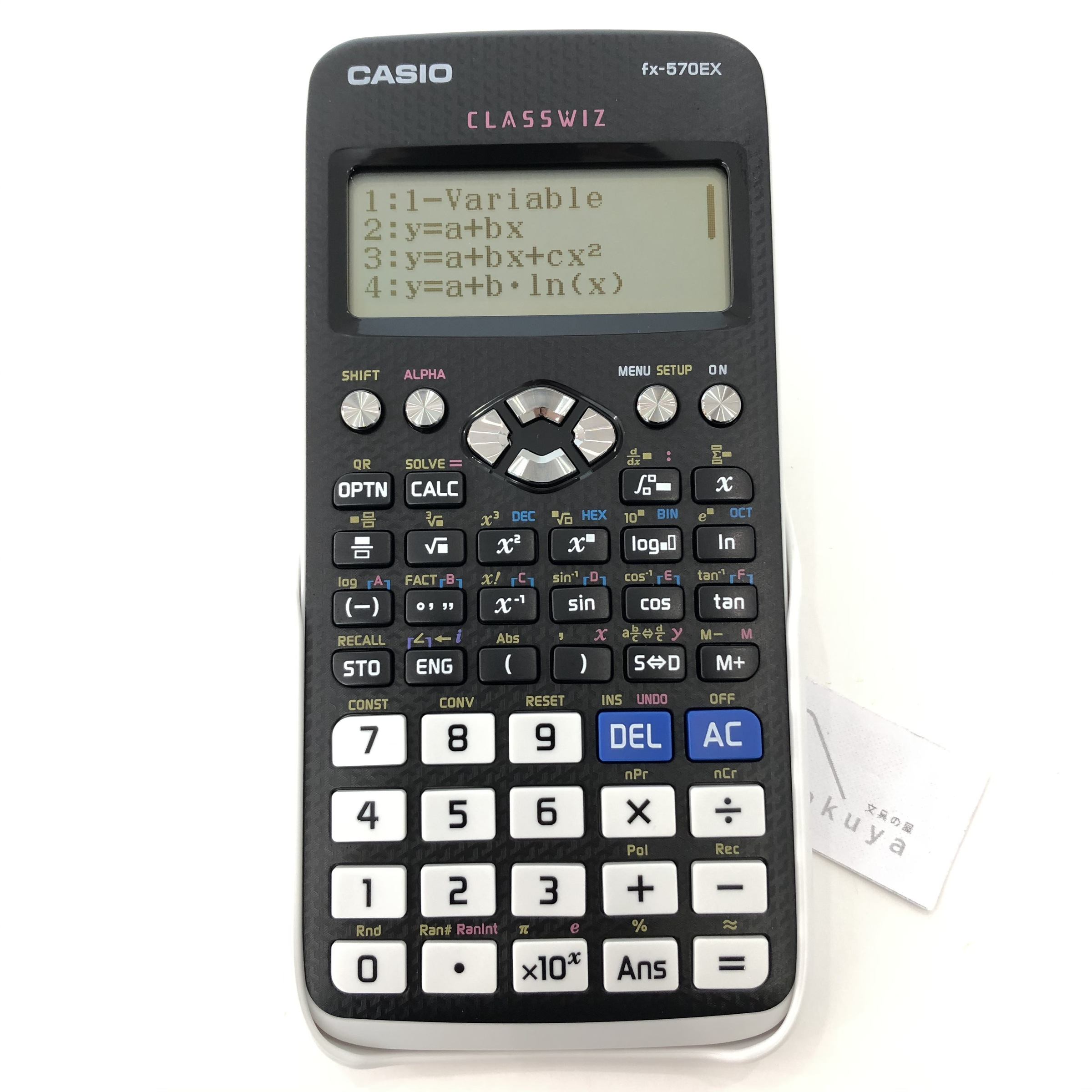•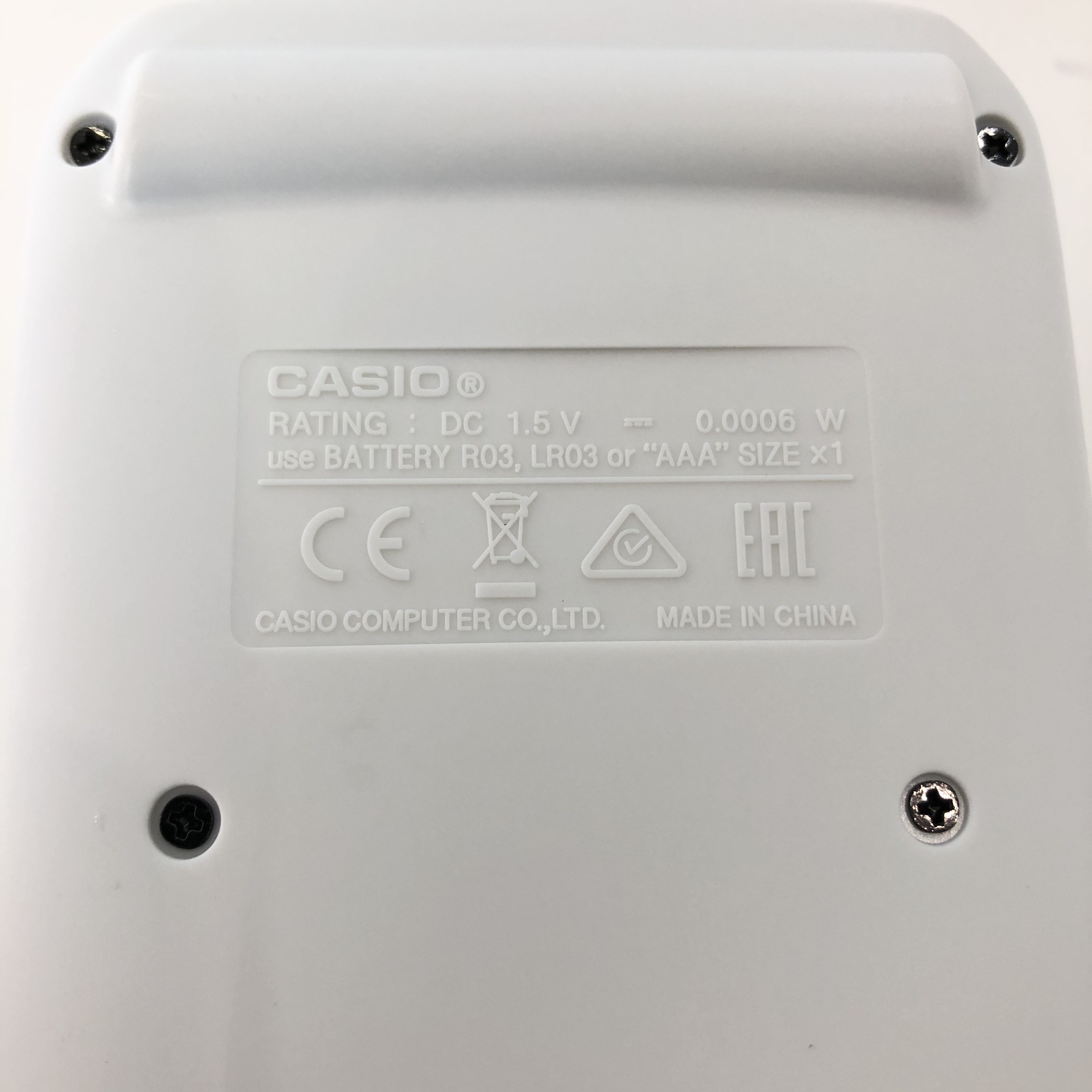CASIO

# CASIO 570EX Scientific Calculator

Regular price
RM 96.00
Sale price
RM 96.00
Regular price
RM 0.00
• Non-programmable, non-graphing calculator.
• GCSE/AS/A Level: Suitable for Cambridge International Examinations GCSE, AS & A Level.
• Number of functions: 552.
• Battery used: AAA (R03) x1.
• Natural textbook display:
• Display expression same as textbook.
• Nearly all English words are displayed on the screen in unabbreviated form.
• Interactive menu display realizes more intuitive operation.
• Specifications:
• 10+2 Digits: 10-digit mantissa + 2-digit exponential display.
• Dot matrix display: High-resolution screen provides beautiful looking graphs every time.
• The use of icons on the menu screen improves viewability.
• Selection of the desired function is quick and easy, by selecting an icon or inputting a number.
• Multi-replay: Quick and easy recall of previously executed formulas for editing and re-execution.
• Plastic keys: Designed and engineered for easy operation.
• Multiline display: Formulas are automatically wrapped to multiple lines in linear output.
• Digit separator: Separation every three digits makes even large numbers easy to read.
• List based STAT-data editor: Viewing and editing of input data in list format, showing data groups (x-data, y-data, frequency) and surrounding data.
• Scientific Constants: You can use constant symbols used in physics, science classes.
• Basic functions:
• Trigonometric
• Exponential logarithmic
• Fraction calculations
• Standard statistics functions such as Mean, SUM, Standard Deviation, and Regression.
• Intermediate Statistics: More statistical functions such as
• Paired-variable statistical calculation
• 4 x 4 matrix calculations
• Calculation of simultaneous equations with four unknowns and quartic equations
• and advanced statistical distribution calculations for speedy and thorough learning .
• Metric conversion function: You can convert a number in one unit to a number in a different unit.
• Vector
• Differentiation
• Matrix calculations
• Equation calculations
• Integrations
• Complex number calculations
• Inequality
• Ratio.
• 9 variable memories.
• QR Code:
• Generate QR codes of equations input into the calculator by a simple operation.
• Graphs and other graphics can be displayed on smartphone or tablet screens.
• Comes with slide-on hard case.
• Source: https://www.casio-intl.com/asia/en/calc/products/fx-570EX/
• Original 1 year local warranty:
• Warranty for buttons & screen under manufacturer defects.
• Unclaimable if item is faulty due to any other Human errors.

Click the links below for more types of scientific calculator: